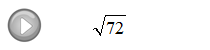Showing posts with label square root. Show all posts
Showing posts with label square root. Show all posts

## Thursday, November 8, 2012

### Solving Quadratic Equations and Graphing Parabolas

So far we have been able to solve quadratic equations using the factoring method.
The idea was to set the equation to zero, factor it, then set each factor to zero and solve. The zero factor property allowed us to solve factorable quadratic equations.

Solve by factoring.
At this point we will learn a new technique that works for quadratic equations that are in the following form:
The idea here is to isolate the x^2 term and then apply the square root property. For example, if
then it is fairly clear that x = -3 or x = 3 which can be gotten using the following property:
When applying the square root to both sides of an equation remember to include the + or -.

Solve by extracting roots:

Step 1: Isolate the square term.
Step 2: Apply the square root property.
Step 3: Be sure to include the + or - and solve as usual.
Compare the next three problems to the first three of this section, they are the same questions. Notice that the two very different techniques give us the same solutions.

Solve by extracting roots.
Solve.

## Saturday, November 3, 2012

### Exponents and Square Roots

To square a number means to multiply that number times itself.  For example:
The number 5 is called the base and the integer 2 is called the exponent.

[ Video Instruction: Exponential Notation ]

Another way to read the above example is "5 raised to the second power."

Simplify.
It is important to point out the last two solved problems, what is different?

The square root can be thought of as the opposite of squaring a number.  In other words, if we want the square root of 16 the question is "what number squared gives 16?"  Actually there are two answers to this question, 4 and −4 because:
Hence, there is a technical distinction here. When we are asked for the square root of numbers we mean the principal (non-negative) square root.

Simplify.
If A and B are positive and real the following property is useful for simplifying square roots:
Look for the largest perfect square factor and the apply this property as follows: